# Reciprocal equation

Determine the root of the equation:

9/x-7/x=1

Correct result:

x =  2

#### Solution: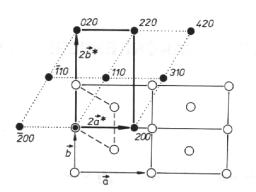We would be pleased if you find an error in the word problem, spelling mistakes, or inaccuracies and send it to us. Thank you!Tips to related online calculators
Do you have a linear equation or system of equations and looking for its solution? Or do you have quadratic equation?

## Next similar math problems:

• Reciprocal equation 3Solve reciprocal equation: 1/2 + 2/3=1/x
• Reciprocal equation 2Solve this equation: x + 5/x - 6 = 4/11
• Eq with reciprocalSolve given equation with reciprocal member: a-6/a+10=4/8
• Equation - inverseSolve for x: 7: x = 14: 1000
• Reciprocal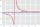It is true (prove it) that if a> b> 0: ?
• Simply equationSolve this equation for x: ?
• Reciprocal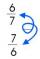For given real numbers, calculate reciprocal number.
• Reciprocal value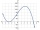How do I calculate a number x that is 9 greater than its reciprocal (1/x)?
• Five tractors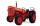Five tractors plow field in 4.8 hours. How long plow this field if after 1.5 hours of work two tractors went to a different field?
• HP - harmonic progressionDetermine the 8th term of the harmonic progression 2, 4/3, 1,…
• One third power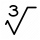Which equation justifies why ten to the one-third power equals the cube root of ten?
• Walnutsx walnuts were in the mission. Dano took 1/4 of nuts Michael took 1/8 from the rest and John took 34 nuts. It stayed here 29 nuts. Determine the original number of nuts.
• Lie/do not lie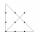The function is given by the rule f(x) = 8x+16. Determine whether point D[-1; 8] lies on this function. Solve graphically or numerically and give reasons for the your answer.
• Determine the number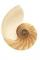Determine the number x that ?.
• Workers9 workers dig a canal 120 meters long for eight hours. For how long would be dig five workers canal 200 meters long?
• Roots and coefficient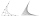In the equation 2x ^ 2 + bx-9 = 0 is one root x1 = -3/2. Determine the second root and the coefficient b.
• Expression with powersIf x-1/x=5, find the value of x4+1/x4